Importance: Medium ✭✭
 Author(s): Atserias, Albert
 Subject: Logic » Finite Model Theory
 Keywords: counting quantifiers FMT12-LesHouches
 Posted by: dberwanger on: October 2nd, 2012
Conjecture   For every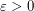there is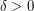such that, for every large, there are-vertex graphsandsuch that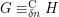and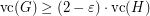.

Here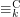denotes indistinguishability in-variable first-order logic with counting quantifiers, and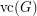denotes the cardinality of the minimum vertex-cover of. By~,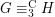implies. Also by~ a positive answer would imply that an integrality gap ofresistslevels of Sherali-Adams linear programming relaxations of vertex-cover, on-vertex graphs. It is known that such a gap resists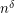levels~. What we ask would let us replaceby. If improving overwere not possible, then we could approximate vertex-cover by a factor better than~in subexponential time (i.e.). Approximating vertex-cover by a factor better than~1.36 is NP-hard~, and approximating vertex-cover by factor better than~2 is UG-hard~, where UG stands for Unique Games (from the Unique Games Conjecture); but note that UG-hardness does not rule out subexponential-time algorithms because UG itself is solvable in subexponential time~

## Bibliography

 A. Atserias and E. Maneva. Sherali-Adams Relaxations and Indistinguishability in Counting Logics, in Proc. 3rd ACM ITCS, pp. 367-379, 2012.

 M. Charikar, K. Makarychev and Y. Makarychev. Integrality Gaps for Sherali-Adams Relaxations, in Proc. 41st ACM STOC, pp. 283-292, 2009.

 I. Dinur and S. Safra. On the Hardness of Approximating Minimum Vertex-Cover, Annals of Mathematics, 162(1):439-485, 2005.

 S. Khot and O. Regev. Vertex cover might be hard to approximate to within 2-epsilon, J. Comput. Syst. Sci. 74(3):335-349, 2008.

 S. Arora, B. Barak, and D. Steurer. Subexponential Algorithms for Unique Games and Related problems, in Proc. 51th IEEE FOCS, pp. 563-572, 2010.}

* indicates original appearance(s) of problem.